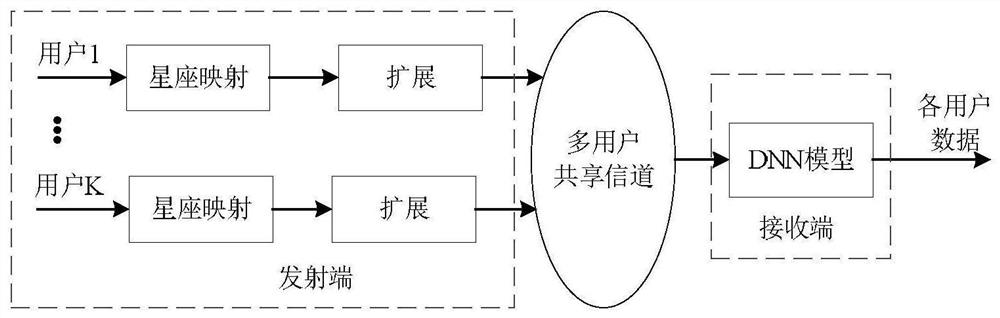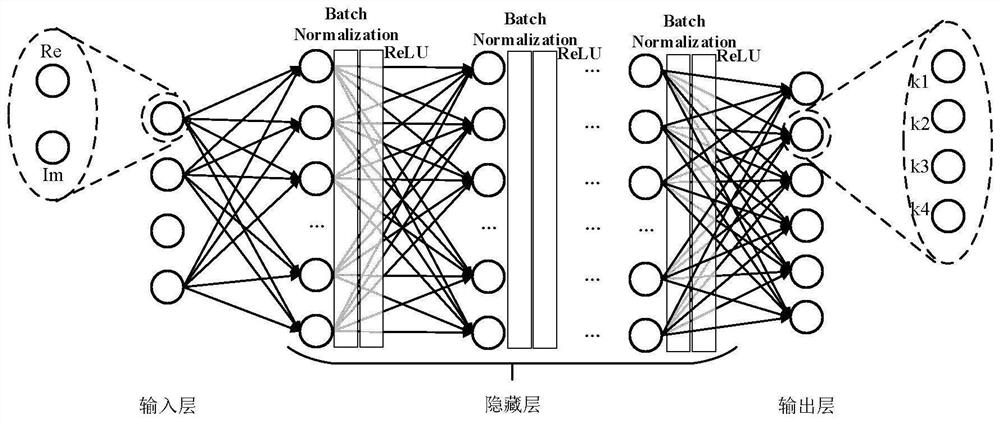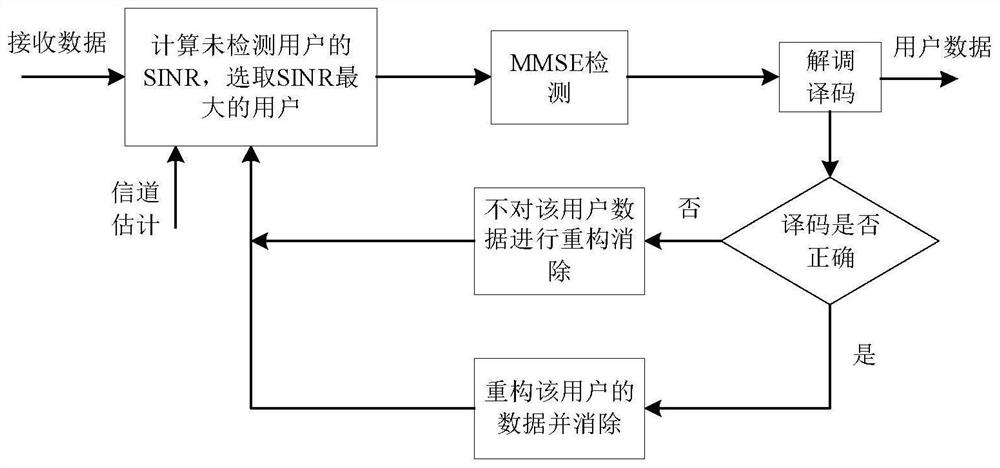# Multi-user shared access receiver based on neural network and communication method thereof

## A neural network and shared access technology, applied in neural learning methods, biological neural network models, neural architectures, etc., can solve problems such as high complexity, receiver bit error rate performance and detection performance need to be improved

Inactive Publication Date: 2021-03-26
HARBIN INST OF TECH +1
4 Cites 0 Cited by

## AI-Extracted Technical Summary

### Problems solved by technology

However, interference cancellation technology often brings high complexity. Considering the excellent performance of deep neural network, neura...
View more

## Abstract

The invention discloses a multi-user shared access receiver based on a neural network and a communication method thereof, relates to the technical field of communication and the field of artificial intelligence, and provides a multi-user shared access receiver better than a traditional MMSE-SIC receiver and a communication method thereof in order to improve the bit error rate performance and detection performance of an existing receiver. The bit error rate and other detection performances of the MMSE-SIC receiver are better than those of a traditional MMSE-SIC receiver no matter under a Gaussian channel or a Rayleigh fading channel.

Application Domain

Neural architecturesTransmission +1

Technology Topic

## Image

•••## Examples

• Experimental program(2)
• Effect test(1)

### Example Embodiment

 DETAILED DESCRIPTION OF THE INVENTION First, a multi-user shared by a neural network shares an access receiver based on a multi-user of a neural network, which includes: DNN model, the DNN model located in the signal receiver, the DNN model including input Layer, hidden layers and output layers;
 The input layer is used to input data of K users;
 The hidden layer is used to hide the data of K users;
 The output layer is used to output data of k users;
 Its characteristics are: the number of hidden layers in the DNN model is L, L is positive integer L = 1, ... L, the L-layer hidden layer contains connection weight matrix W l , Bias vector B l And activation function σ l , The neurons of each layer use the same σ l The structure of the DNN model is expressed as:
 σ L (W L σ L-1 (... σ 1 (W 1 X + B 1 ) ...) + b L ),
 Where: x represents the input to be processed; ie: the output z of the DNN network model is represented as:
 z = σ L (W L σ L-1 (Lσ) 1 (W 1 X + B 1 ) + B L )
 The above formula is an output data obtained by inputting data R before the forward propagation of the DNN network model.

### Example Embodiment

 In particular, a multi-user shared by a neural network according to a particular embodiment is a communication method of incoming receiver: its feature: its signal emission method:
 Step 1, K users perform the constellation map, obtain K map results;
 Step 2, the K mapping results obtained by the steps are respectively extended, and k is obtained, respectively, and obtain K extended processing results;
 Step 3, the K extended processing result obtained by the step is transmitted to a multi-user sharing channel as the transmit signal;
 Its signal receiving method: The receiving end is processed in the multi-user shared channel to receive the transmit signal transmitted by the transmitting end and sent into the DNN model;
 Step 4, the DNN model outputs the original data of K users, completes communication with multiple users based on neural networks. Communication.
 Principle: a) MMSE-SIC detection algorithm
 The MMSE-SiC algorithm used by the receiving end of MUSA. It mainly includes three steps of sorting, detection, and interference cancellation, and the flow block diagram image 3 Indicated. The processing steps obtained by the drawing are as follows:
 (1) The equivalent channel coefficient is calculated by the extended sequence W selected by the channel coefficient h and the user;
 (2) Calculate the SINR of the remaining detection user using the equivalent channel coefficient f and MMSE, and sort by size, and the calculation of SINR is shown below;

 (3) Select SINR's largest user to utilize multiplication MMSE detection, then the user's data estimation can be obtained by demodulation decoding;
 (4) If the user data can be reconstructed, the user data can be reconstructed, and the reconstructed data can be regarded as the interference to be detected, so it is necessary to subtract the interference from the received signal, the decoding error is not performed. Reconstructing the elimination operation.
 (5) Repeat (2) - (4) step until all users' data is completely detected.
 It can be seen from the above analysis, the algorithm needs to calculate the SINR of each user and sorted, involving the retrieval of multiple matrices, and only detects only one user's data, the number of users increases, the processing time delay and processing complexity will change Big. Therefore, scholars at home and abroad are optimized for user sorting and interference cancellation technology to balance between detection performance and detection complexity. However, these conventional detection algorithms are difficult to enhance substantial improvement in MUSA's detection performance.
 B), deep neural network
 In recent years, with the rapid development of ML and DL technology, many scholars have begun to apply these technologies to a broader field, while having good performance improvements in many areas including communication technology. Considering that DNN technology has strong nonlinear fitting capabilities, many nonlinear complex problems can be solved, and the network structure of DNN is flexible and easy to adjust, so this article applies DNN technology to MUSA multi-user detection.
 First, DNN technology is introduced, and multiple neurons can be configured to have a DNN network having a multi-layer neural structure, and a network structure. Figure 4 Indicated.
 by Figure 4 It can be seen that the DNN structure includes the input layer of the data input, the hidden layer of the data operation, and the output layer of the final data output, and the hidden layer is usually included in the case. Each layer of DNN also includes multiple neurons. Further, DNN is a fully connected network, ie, the neuron of the layer is connected to the upper layer or the neuron of the next layer. Suppose the DNN network contains L hidden layers, then the L-layer hidden layer contains the connection weight matrix W l , Bias vector B l And activation function σ l , The neurons of each layer use the same σ l. In this case, the DNN structure can be expressed as σ L (W L σ L-1 (... σ 1 (W 1 X + B 1 ) ...) + b L ), Where x represents input to be processed. That is, the output z of the DNN network can be expressed as
 z = σ L (W L σ L-1 (Lσ) 1 (W 1 X + B 1 ) + B L )
 The above formula can be considered as an output obtained by input R passing through the DNN.
 In a supervised learning, the purpose of the DNN network is to make the input of sample data as close as possible to the output of sample data. For example, there is a training sample: { 1 Y 1 ), (x 2 Y 2 ), L, (X m Y m ), L, (X M Y M )}, Through this M sample training DNN network, then use the training of M's network model to predict new test sample X test Output. The training DNN network process first sets the initial value of the DNN parameter θ, the weight and the offset, and then obtains the training sample data input through the DNN network to the output of the propagation as shown in the above formula, and then design the appropriate loss function metrics DNN output Z and The distance between the sample data output Y, in order to minimize the gap between Z and Y, the reverse propagation of the DNN network is constantly correcting the DNN parameter θ until the loss function satisfies the requirements. The process of training can be expressed as

 The DNN model constructed in the final use of the training parameter θ can predict the output of the new test sample, the predicted process is the output of the DNN forward propagation by DNN.
 Applying DNN to MUSA multi-user and detection, the DNN structure can be utilized as a receiver of the MUSA system, and then the sample data generated after the conventional MUSA transmitter is added to the channel condition as DNN training data, the sample is trained to receive signals of the receiver. As the input of the sample, the original user signal of the receiver receive signal is formed as the output of the sample. After the DNN network training is completed, the model after the training can realize the receiver receiving signal input under different channel conditions, i.ey the user signal forming the received signal, thereby realizing a multi-user detection of the MUSA receiver received signal. MUSA system DNN multi-user testing scheme figure 1 Indicated.
 In the DNN multi-user detection scheme of the MUSA system, the transmitting end still uses the MUSA traditional transmitter. The signals of each user first completed the constellation mapping by QPSK modulation, and then you can get the modulation symbols of each user, then these modulation symbols need to be extended sequences. During expansion, then the data of each user can be transmitted over a multi-user shared channel. The receiving end no longer uses the MUSA conventional MMSE-SIC receiver, but directly using the DNN model as the MUSA receiver to complete the multi-user detection of the MUSA receiving end to obtain raw data of each user.
 The DNN multi-user detecting the DNN network model of the DNN network model of the DNN multi-user in this article is like Figure 4 As shown, the specific structure is directed to the MUSA system at the same time, and the extended sequence of length 4 is used, that is, the user's overload rate is 150%. For other overload ratios, the number of neurons of the input layer and the output layer needs to be adjusted.
 It can be seen from the above analysis, the algorithm needs to calculate the SINR of each user and sorted, involving the retrieval of multiple matrices, and only detects only one user's data, the number of users increases, the processing time delay and processing complexity will change Big. Therefore, scholars at home and abroad are optimized for user sorting and interference cancellation technology to balance between detection performance and detection complexity. However, these conventional detection algorithms are difficult to enhance substantial improvement in MUSA's detection performance.
 C), deep neural network
 In recent years, with the rapid development of ML and DL technology, many scholars have begun to apply these technologies to a broader field, while having good performance improvements in many areas including communication technology. Considering that DNN technology has strong nonlinear fitting capabilities, many nonlinear complex problems can be solved, and the network structure of DNN is flexible and easy to adjust, so this article applies DNN technology to MUSA multi-user detection.
 First, DNN technology is introduced, and multiple neurons can be configured to have a DNN network having a multi-layer neural structure, and a network structure. Figure 4 Indicated.
 by Figure 4 It can be seen that the DNN structure includes the input layer of the data input, the hidden layer of the data operation, and the output layer of the final data output, and the hidden layer is usually included in the case. Each layer of DNN also includes multiple neurons. Further, DNN is a fully connected network, ie, the neuron of the layer is connected to the upper layer or the neuron of the next layer. Suppose the DNN network contains L hidden layers, then the L-layer hidden layer contains the connection weight matrix W l , Bias vector B l And activation function σ l , The neurons of each layer use the same σ l. In this case, the DNN structure can be expressed as σ L (W L σ L-1 (... σ 1 (W 1 X + B 1 ) ...) + b L ), Where x represents input to be processed. That is, the output z of the DNN network can be represented as:
 z = σ L (W L σ L-1 (Lσ) 1 (W 1 X + B 1 ) + B L )
 The above formula can be considered as an output obtained by input R passing through the DNN.
 In a supervised learning, the purpose of the DNN network is to make the input of sample data as close as possible to the output of sample data. For example, there is a training sample: { 1 Y 1 ), (x 2 Y 2 ), L, (X m Y m ), L, (X M Y M )}, Through this M sample training DNN network, then use the training of M's network model to predict new test sample X test Output. The training DNN network process first sets the initial value of the DNN parameter θ, the weight and the offset, and then obtains the training sample data input through the DNN network to the output of the propagation as shown in the above formula, and then design the appropriate loss function metrics DNN output Z and The distance between the sample data output Y, in order to minimize the gap between Z and Y, the reverse propagation of the DNN network is constantly correcting the DNN parameter θ until the loss function satisfies the requirements. The process of training can be expressed as

 The DNN model constructed in the final use of the training parameter θ can predict the output of the new test sample, the predicted process is the output of the DNN forward propagation by DNN.
 The workflow of the entire system is as follows:

 V. Inventive effect:
 By this method, you can design a multi-user sharing access DNN receiver than the traditional MMSE-SIC receiver. Its error rate and other detection performance are better than traditional MMSE-SiC receivers under the Gaussian fading channel.
 This method is simple, the computational complexity is low, easy to implement.

## PUM## Description & Claims & Application Information

We can also present the details of the Description, Claims and Application information to help users get a comprehensive understanding of the technical details of the patent, such as background art, summary of invention, brief description of drawings, description of embodiments, and other original content. On the other hand, users can also determine the specific scope of protection of the technology through the list of claims; as well as understand the changes in the life cycle of the technology with the presentation of the patent timeline. Login to view more.
Who we serve
• R&D Engineer
• R&D Manager
• IP Professional
Why Eureka